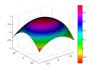# Equation with two second order variables

• dunkdie
In summary, the conversation discusses the discovery of a helpful community forum and a college project involving an equation for a surface graph. The person is looking for a method to find the values of X and Y when given a value for Z. The conversation also raises questions about the accuracy of the method used to derive the equation for the surface graph. The attempt at a solution involves using the differential method, but no solution has been found yet.

#### dunkdie

Hi all,

I've come across this forum when searching for solution to my problem and I found that the community is extremely helpful :)

I was working on my college project when I came across the equation below.

## Homework Statement

Z = -0.0006*X^2 + 0.0004*X -0.0008*Y^2 - 0.0006*Y + 0.956

Suppose that I know the value of Z (as example Z=0.15), which method can I use to find the value of X and Y?

## Homework Equations

The equation above was derived when I was trying to chart a surface graph from these two equations:

Z = -0.0006*X^2 + 0.0004*X + 0.956

and

Z = -0.0008*Y^2 - 0.0006*Y + 0.956

However I don't know the correctness of this method that I used to come up with surface graph Z = -0.0006*X^2 + 0.0004*X -0.0008*Y^2 - 0.0006*Y + 0.956
The picture of this surface graph is attached.

## The Attempt at a Solution

I tried using differential method to solve the equation but so far no luck :( Hope somebody would come up with some method or explanation regarding the matter. Many thanks.

Regards,
John

#### Attachments

•untitled.png
12.6 KB · Views: 357
For a given value of z, your equation determines an ellipse. Complete the square in the x and y terms to get the ellipse into standard form.

Z = -0.0006*X^2 + 0.0004*X -0.0008*Y^2 - 0.0006*Y + 0.956

You said it came about from these equations:
Z = -0.0006*X^2 + 0.0004*X + 0.956
Z = -0.0008*Y^2 - 0.0006*Y + 0.956

Presumably you set the two right sides equal, which would give you

-0.0006*X^2 + 0.0004*X + 0.956 = -0.0008*Y^2 - 0.0006*Y + 0.956
or, equivalently, -0.0006*X^2 + 0.0004*X = -0.0008*Y^2 - 0.0006*Y

Notice that there is no z and no 0.956.

The equation above represents a hyperbola that is the intersection of the to parabolic cylinders Z = -0.0006*X^2 + 0.0004*X + 0.956 and Z = -0.0008*Y^2 - 0.0006*Y + 0.956.